Next: Is there an efficient Up: Convex Polyhedron Previous: How many facets can   Contents

## How hard is it to verify that an H-polyhedronand a V-polyhedron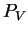are equal?

This is a fundamental complexity question associated with the Minkowski-Weyl theorem (Theorem 5). This problem, known as the polyhedral verification problem was first posed by L. Lovasz (see [Sey94]).

To simplify our discussion, let us assumeandare bounded and thus polytopes. Also we may assume that the given representations contain no redundant data, since removing redundancies is just a matter of solving linear programs, see Sections 2.19 and 2.21.

The verification consists of two questions, ``is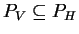?'' and ``is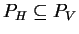?'' The first question is easy to answer by just checking whether each generator (vertex) ofsatisfies the H-representation of. The second question is known to be coNP-complete, due to [FO85]. (It is not hard to see that it belongs to coNP, since the negative answer to the question has a succinct certificate, a vertex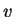ofand a hyperplane separatingfrom.) Yet, the complexity of the second question, when the first question has the positive answer, is not known.

It is possible to prove that the polynomial solvability of this problem implies the polynomial solvability of the representation conversion problem for general convex polytopes (i.e. the vertex enumeration and the facet enumeration problems). Here the polynomial solvability of the representation conversion problem means the existence of an algorithm that generates the second minimal representation in time polynomial in the size of both input and output. See Section 2.15 for discussion on complexity measures.

How does the above reduction work? Assume we have a polynomial algorithm for the verification, and we design an algorithm to generate all vertices of an H-polytope. Let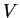be a set of vertices ofgenerated so far. Take the first inequality from the H-representation, and ask whether we have generated all vertices on the face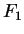, the intersection ofand the hyperplane given by the first inequality being forced to be equality. This is just one application of the verification algorithm. If yes, we move to the second inequality and repeat. Otherwise, we go down to lower dimensional face by setting one of the remaining inequality to equality. When we have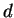-independent equalities, we compute the unique vertex by solving the equation system. The key observation is that we generate a subproblem only when the verification algorithm returns NO answer. This means every subproblem created generates at least one new vertex. This guarantees our generation algorithm to be polynomial.

I do not know who is the first to recognize this reduction. I consider this belongs to folklore.

Finally I repeat: the complexity of the polyhedral verification problem is unknown. Is it in P or in coNP-complete? This is perhaps the most important question in polyhedral computation. A fascinating question, indeed.Next: Is there an efficient Up: Convex Polyhedron Previous: How many facets can   Contents
Komei Fukuda 2004-08-26